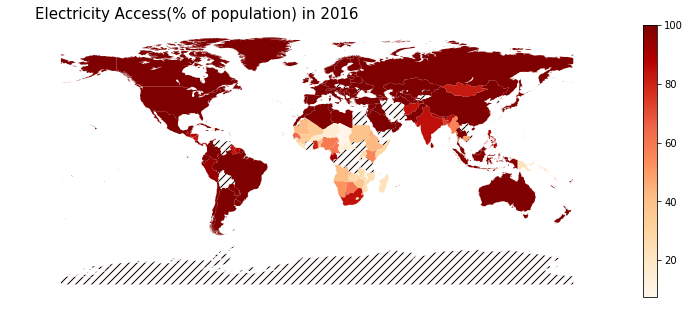# Visualising Electricity Access Over Space and Time

How is the world changing over the years!
sustainability
Author

Nipun Batra

Published

June 26, 2018

In this post, I’ll explore electricity access, i.e. globally what fraction of people have access to electricity. Beyond the goal of finding the electricity access, this post will also serve to illustrate how the `coolness` coefficient of the Python visualisation ecosystem!

I’ll be using data from World Bank for electricity access. See the image below for the corresponding page.Now, a Python package called `wbdata` provides a fairly easy way to access World Bank data. I’d be using it to get data in Pandas `DataFrame`.

``````%matplotlib inline
import pandas as pd
import wbdata
import matplotlib.pyplot as plt
import datetime
data_date = (datetime.datetime(1990, 1, 1), datetime.datetime(2016, 1, 1))
df_elec = wbdata.get_data("EG.ELC.ACCS.ZS", pandas=True, data_date=data_date)``````
``df_elec.head()``
``````country     date
Arab World  2016    88.768654
2015    88.517967
2014    88.076774
2013    88.389705
2012    87.288244
Name: value, dtype: float64``````

I’d now be downloading `shapefile` data for different countries. This will help us to spatially plot the data for the different countries.

``!wget http://naciscdn.org/naturalearth/10m/cultural/ne_10m_admin_0_countries_lakes.zip``
``````--2018-06-26 15:52:50--  http://naciscdn.org/naturalearth/10m/cultural/ne_10m_admin_0_countries_lakes.zip
Resolving naciscdn.org (naciscdn.org)... 146.201.97.163
Connecting to naciscdn.org (naciscdn.org)|146.201.97.163|:80... connected.
HTTP request sent, awaiting response... 200 OK
Length: 5077755 (4.8M) [application/x-zip-compressed]

ne_10m_admin_0_coun 100%[===================>]   4.84M   246KB/s    in 22s

2018-06-26 15:53:12 (228 KB/s) - ‘ne_10m_admin_0_countries_lakes.zip’ saved [5077755/5077755]
``````

#### Extracting shapefile

``````import zipfile
zip_ref.extractall('.')
zip_ref.close()``````
``````import geopandas as gpd
``gdf.head()``
0 IDN (POLYGON ((117.7036079039552 4.163414542001791...
1 MYS (POLYGON ((117.7036079039552 4.163414542001791...
2 CHL (POLYGON ((-69.51008875199994 -17.506588197999...
3 BOL POLYGON ((-69.51008875199994 -17.5065881979999...
4 PER (POLYGON ((-69.51008875199994 -17.506588197999...

### Visualising electricity access in 2016

#### Getting electricity access data for 2016

``df_2016 = df_elec.unstack()[['2016']].dropna()``
``df_2016.head()``
date 2016
country
Afghanistan 84.137138
Albania 100.000000
Algeria 99.439568
Andorra 100.000000
Angola 40.520607

In order to visualise electricity access data over the map, we would have to join the GeoPandas object `gdf` and `df_elec`

#### Joining `gdf` and `df_2016`

Now, `gdf` uses `alpha_3` codes for country names like AFG, etc., whereas `df_2016` uses country names. We will thus use `pycountry` package to get code names corresponding to countries in `df_2016` as shown in this StackOverflow post.

``````import pycountry
countries = {}
for country in pycountry.countries:
countries[country.name] = country.alpha_3
codes = [countries.get(country, 'Unknown code') for country in df_2016.index]
df_2016['Code'] = codes``````
``df_2016.head()``
date 2016 Code
country
Afghanistan 84.137138 AFG
Albania 100.000000 ALB
Algeria 99.439568 DZA
Andorra 100.000000 AND
Angola 40.520607 AGO

Now, we can join the two data sources

``merged_df_2016 = gpd.GeoDataFrame(pd.merge(gdf, df_2016, left_on='ADM0_A3', right_on='Code'))``
``merged_df_2016.head()``
0 IDN (POLYGON ((117.7036079039552 4.163414542001791... 97.620000 IDN
1 MYS (POLYGON ((117.7036079039552 4.163414542001791... 100.000000 MYS
2 CHL (POLYGON ((-69.51008875199994 -17.506588197999... 100.000000 CHL
3 PER (POLYGON ((-69.51008875199994 -17.506588197999... 94.851746 PER
4 ARG (POLYGON ((-68.4486097329999 -52.3466170159999... 100.000000 ARG

#### Finally plotting!

``````# Example borrowed from http://ramiro.org/notebook/geopandas-choropleth/
cmap='OrRd'
figsize = (16, 5)
ax = merged_df_2016.plot(column='2016', cmap=cmap, figsize=figsize,legend=True)
title = 'Electricity Access(% of population) in {}'.format('2016')
ax.set_title(title, fontdict={'fontsize': 15}, loc='left')
ax.set_axis_off()``````### Creating animation for access across time

``!mkdir -p elec_access``
``````def save_png_year(year, path="elec_access"):
df_year = df_elec.unstack()[['{}'.format(year)]].dropna()
codes = [countries.get(country, 'Unknown code') for country in df_year.index]
df_year['Code'] = codes
merged_df_year = gpd.GeoDataFrame(pd.merge(gdf, df_year, left_on='ADM0_A3', right_on='Code'))
figsize = (16, 5)
ax = merged_df_year.plot(column='{}'.format(year), cmap=cmap, figsize=figsize,legend=True,vmin=0.0, vmax=100.0)
title = 'Electricity Access(% of population) in {}'.format(year)
ax.set_title(title, fontdict={'fontsize': 15}, loc='left')
ax.set_axis_off()
plt.savefig('{}/{}.png'.format(path, year), dpi=300)
plt.close()``````
``````for year in range(1990, 2017):
save_png_year(year)``````
``````# Borrowed from http://www.kevinwampler.com/blog/2016/09/10/creating-animated-gifs-using-python.html
def create_gifv(input_files, output_base_name, fps):
import imageio
output_extensions = ["gif"]
input_filenames = ['elec_access/{}.png'.format(year) for year in range(1990, 2017)]

poster_writer = imageio.get_writer("{}.png".format(output_base_name), mode='i')
video_writers = [
imageio.get_writer("{}.{}".format(output_base_name, ext), mode='I', fps=fps)
for ext in output_extensions]

is_first = True
for filename in input_filenames:

for writer in video_writers:
writer.append_data(img)
if is_first:
poster_writer.append_data(img)

is_first = False

for writer in video_writers + [poster_writer]:
writer.close()``````
``create_gifv("elec_access/*.png", "electricity_access", 4)``Across Africa and SE Asia, one can clearly see a gradual improvement in access! Hope you had fun reading this post :)